GeeksforGeeks App
Open AppBrowser
Continue

## Related Articles

• RD Sharma Class 8 Solutions for Maths

# Class 8 RD Sharma Solutions- Chapter 20 Area Of Trapezium And Polygon- Exercise 20.1 | Set 1

### Question 1. A flooring tile has the shape of a parallelogram whose base is 24 cm and the corresponding height is 10 cm. How many such tiles are required to cover a floor of area 1080 m2?

Solution:

Given that,

Base of parallelogram = 24cm,

Height of parallelogram = 10cm,

Area of floor = 1080m2

As we know that,

Area of parallelogram = Base × Height

Area of 1 tiles = 24 × 10 = 240 cm2

As we know that 1m = 100cm,

So area will be 1080 m2 = 1080 × 100 × 100 cm2

Number of tiles required = Area of floor / Area of 1 tile

Number of tiles required = (1080 × 100 × 100) / (24 × 10) = 45000

Hence, Number of tiles required is 45000.

### Question 2. A plot is in the form of a rectangle ABCD having semi-circle on BC as shown in Figure. If AB = 60 m and BC = 28 m, Find the area of the plot.

Solution: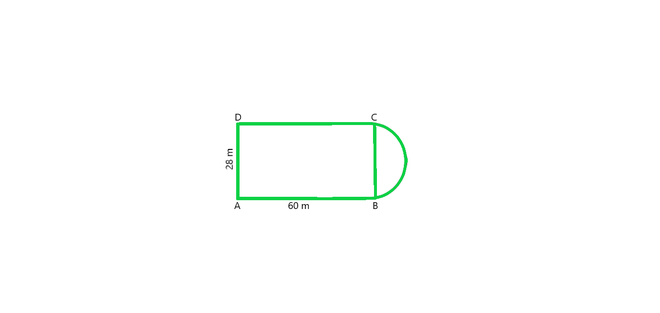From figure, we conclude that, Area of the plot = Area of the rectangle + Area of semi-circle

Radius of semi-circle = BC/2 = 28/2 = 14m

As we know that Area of the Rectangular plot = Length × Breadth = 60 × 28 = 1680 m2

Area of the Semi-circular portion = πr2/2

= 1/2 × 22/7 × 14 × 14 = 308 m2

Hence, the total area of the plot = 1680 + 308 = 1988 m2

### Question 3. A playground has the shape of a rectangle, with two semi-circles on its smaller sides as diameters, added to its outside. If the sides of the rectangle are 36 m and 24.5 m, find the area of the playground. (Take π= 22/7.)

Solution: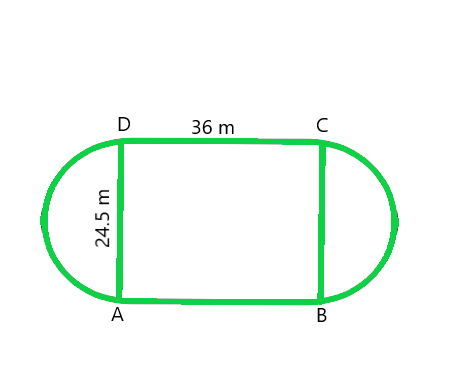From figure, we conclude that, Area of the plot = Area of the Rectangle + 2 × area of one semi-circle

Radius of semi-circle = BC/2 = 24.5/2 = 12.25m                                (Given)

As we know that Area of the Rectangular plot = Length × Breadth = 36 × 24.5 = 882 m2

and Area of the Semi-circular portions = 2 × πr2/2

= 2 × 1/2 × 22/7 × 12.25 × 12.25 = 471.625 m2

Hence, the total Area of the plot = 882 + 471.625 = 1353.625 m2

### Question 4. A rectangular piece is 20 m long and 15 m wide. From its four corners, quadrants of radii 3.5 m have been cut. Find the area of the remaining part.

Solution: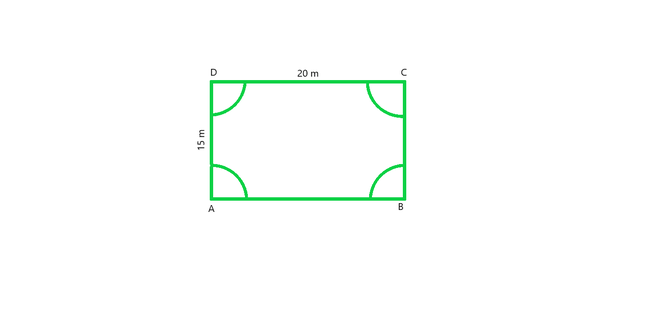From figure, we conclude that, Area of the plot = Area of the rectangle – 4 × area of one quadrant

Radius of semi-circle = 3.5 m                                        (Given)

Area of four quadrants = area of one circle                        (from figure)

Area of the plot = Length × Breadth – πr2

Hence, Area of the plot = 20 × 15 – (22/7 × 3.5 × 3.5) = 261.5 m2

### Question 5. The inside perimeter of a running track (shown in Fig. 20.24) is 400 m. The length of each of the straight portion is 90 m and the ends are semi-circles. If track is everywhere 14 m wide, find the area of the track. Also, find the length of the outer running track.

Solution: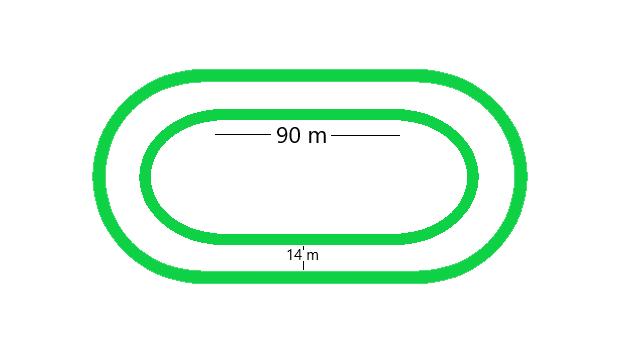From figure, we conclude that, Perimeter of the inner track = 2 × Length of rectangle + perimeter of two semi-circular ends

Perimeter of the inner track = Length + Length + 2πr

400 = 90 + 90 + (2 × 22/7 × r)

(2 × 22/7 × r) = 400 – 180

(2 × 22/7 × r) = 220

44r = 220 × 7

44r = 1540

r = 1540/44 = 35

r = 35m

Hence, the radius of inner circle = 35 m

Now we have to calculate the radius of outer track

Radius of outer track = Radius of inner track + width of the track                                     (from figure)

Radius of outer track = 35 + 14 = 49m                                   (given)

Length of outer track = 2× Length of rectangle + perimeter of two outer semi-circular ends

Length of outer track = 2× 90 + 2πr

Length of outer track = 2× 90 + (2 × 22/7 × 49)

Length of outer track = 180 + 308 = 488

Hence, the Length of outer track = 488m

Area of inner track = Area of inner rectangle + Area of two inner semi-circles

Area of inner track = Length × Breadth + πr2

Area of inner track = 90 × 70 + (22/7 × 35 × 35)

Area of inner track = 6300 + 3850

Hence, the Area of inner track = 10150 m2

Area of outer track = Area of outer rectangle + Area of two outer semi-circles

Breadth of outer track = 35 + 35 +14 + 14 = 98 m

Area of outer track = length× breadth + πr2

Area of outer track = 90 × 98 + (22/7 × 49 × 49)

Area of outer track = 8820 + 7546

Hence, Area of outer track = 16366 m2

Now, we have to calculate the Area of path

Area of path = Area of outer track – Area of inner track

Area of path = 16366 – 10150 = 6216

Hence, Area of path is 6216 m2

### Question 6. Find the area of Fig., in square cm, correct to one place of decimal. (Take π =22/7)

Solution: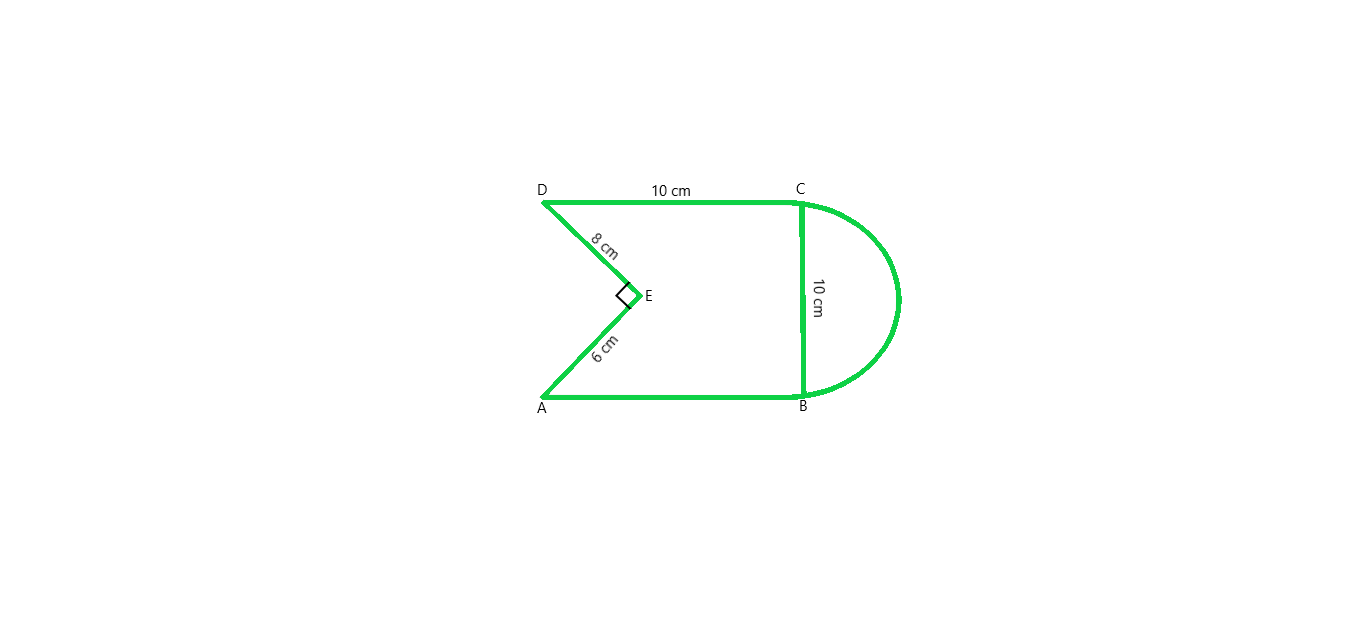From figure we conclude that, Area of the Figure = Area of square + Area of semi-circle – Area of right angled triangle

Area of the Figure = side × side + πr2/2 – (1/2 × base × height)

put the values in formula and we get,

Area of the Figure = 10 × 10 + (1/2 × 22/7 × 5 × 5) – (1/2 × 8 × 6)

Area of the Figure = 100 + 39.28 – 24

Area of the Figure = 115.3

Hence, Area of the Figure = 115.3 cm2

### Question 7. The diameter of a wheel of a bus is 90 cm which makes 315 revolutions per minute. Determine its speed in kilometres per hour. (Take π=22/7)

Solution:

Given that,

Diameter of a wheel = 90 cm,

As we know that, Perimeter of wheel = πd

Perimeter of wheel = 22/7 × 90 = 282.857

Hence, the Perimeter of a wheel = 282.857 cm

Distance covered in 315 revolutions = 282.857× 315 = 89099.955 cm

As we know that 1 km = 100000 cm

So, The Distance covered = 89099.955/100000 = 0.89 km

Speed in km per hour = 0.89 × 60 = 53.4 km per hour.

### Question 8. The area of a rhombus is 240 cm^2 and one of the diagonal is 16 cm. Find another diagonal.

Solution:

Given that,

Area of rhombus = 240 cm^2,

Diagonal = 16 cm.

As we know that,

Area of rhombus = 1/2 × d1 × d2

240 = 1/2 × 16 × d2

240 = 8 × d2

d2 = 240/8 = 30

Hence the other diagonal is 30 cm.

### Question 9. The diagonals of a rhombus are 7.5 cm and 12 cm. Find its area.

Solution:

Given that,

Diagonal(d1) = 7.5 cm,

Diagonal(d2) = 12 cm.

As we know that,

Area of rhombus = 1/2 × d1 × d2

Area of rhombus = 1/2 × 7.5 × 12

Area of rhombus = 6 × 7.5 = 45

Hence, Area of rhombus = 45 cm2

### Question 10. The diagonal of a quadrilateral shaped field is 24 m and the perpendiculars dropped on it from the remaining opposite vertices are 8 m and 13 m. Find the area of the field.

Solution

As we know that,

Area of quadrilateral = 1/2 × d1 × (p1 + p2)

Area of quadrilateral = 1/2 × 24 × (8 + 13)

Area of quadrilateral = 12 × 21 = 252

Hence, Area of quadrilateral is 252 cm2

### Question 11. Find the area of a rhombus whose side is 6 cm and whose altitude is 4 cm. If one of its diagonals is 8 cm long, find the length of the other diagonal.

Solution:

Given that,

Side of rhombus = 6 cm,

Altitude of rhombus = 4 cm.

As we know that rhombus is a parallelogram, therefore area of parallelogram = base × altitude

Therefore, Area of parallelogram = 6 × 4 = 24 cm2

Area of parallelogram = Area of rhombus

Area of rhombus = 1/2 × d1 × d2

24 = 1/2 × 8 × d2

24 = 4 × d2

d2 = 24/4 = 6

Hence, the length of other diagonal of rhombus is 6 cm.

### Chapter 20 Area Of Trapezium And Polygon- Exercise 20.1 | Set 2

My Personal Notes arrow_drop_up
Related Tutorials